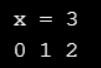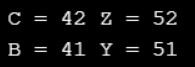# Enum in C

## Enum in C Language with Examples

In this article, I am going to discuss the Enum in C Language with Examples. Please read our previous articles, where we discussed the Command Line Arguments in C. As part of this article, you will learn what is Enum in C, and when and how to use Enum in C Program with examples.

##### Enum in C:

Enum is a keyword, by using this keyword we can create a sequence of integer constant value. Generally, by using enum, we can create a user-defined data type of integer. The size of enumerator datatype is 2B & the range from -32768 to 32767. It is possible to change enum related variable value but it is not possible to change enum constant data.

Syntax: enum tagname {const1=value, const2=value, const3=value};

According to syntax, if const1 value is not initialized, then by default sequence will start from 0 and the next generated value is previous const value+1.

##### Program to understand Enum in C Language:
```#include<stdio.h>
enum ABC{ A, B, C };
int main ()
{
enum ABC x;
x = A + B + C;
printf ("\n x = %d", x);
printf ("\n %d %d %d", A, B, C);
return 0;
}
```
###### Output:##### Program to understand Enum:
```#include<stdio.h>
#include<conio.h>
enum month { Jan = 1, Feb, Mar };
int main ()
{
enum month April;
April = Mar + 1;
printf ("\n %d %d ", Feb, April);
getch ();
return 0;
}
```

Output: 2 4

In the previous program, enum month is a user-defined data type of int, April is variable of type enum month. Enum related constant values are not allowed to modify, enum related variables are possible to modify.

##### Program to understand Enum in C Language:
```#include<stdio.h>
#include<conio.h>
typedef enum ABC { A = 40, B, X = 50, Y } XYZ;
int main ()
{
enum ABC C;
XYZ Z;
C = B + 1;
Z = Y + 1;
printf ("\n C = %d Z = %d", C, Z);
printf ("\n B = %d Y = %d", B, Y);
getch ();
return 0;
}
```
###### Output:In previous program enum ABC is a user-defined data type of integer, XYZ is an alias name to enum ABC.